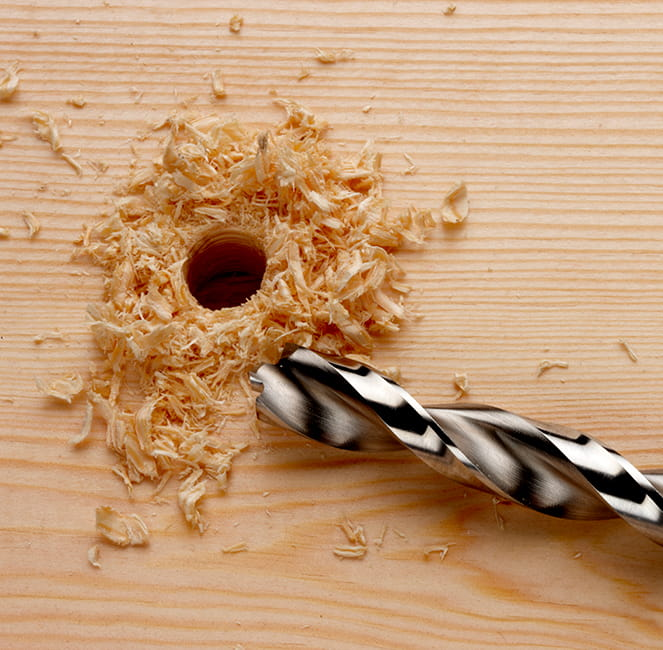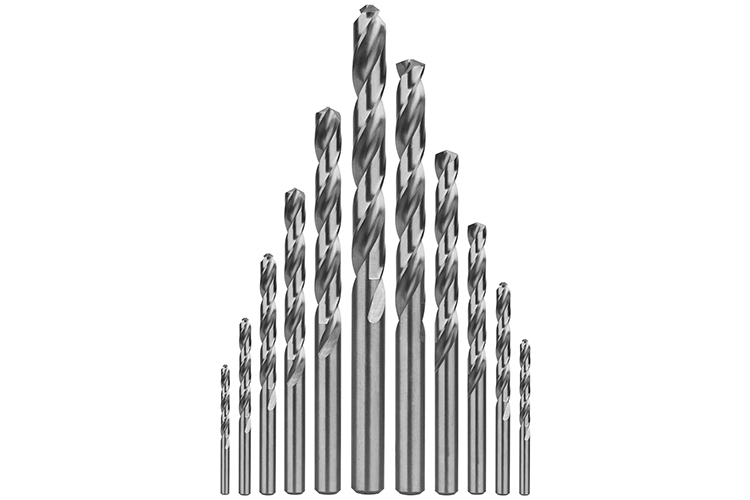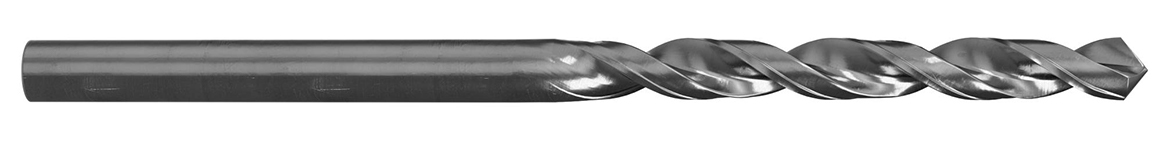Many types of screws require that a pilot hole be drilled before they are installed. Self-drilling screws can, of course, drill their own pilot hole, but other types of tapping, thread cutting, thread forming or thread rolling screws need help getting started. Blunt tipped screws like Type B Tapping, Type F Thread Cutting & Thread Forming Screws for Plastics or Metals require the proper sizing to allow for insertion into material. Even sharp pointed screws such as Type A or Type AB Self-Tapping Screws can split wood or distort metal as they're driven if they don't have a properly sized pilot hole. Below, we have included charts displaying pilot hole diameter and proper drill bit sizing for most varieties of screws we carry. At the end of this section, we've also included a chart showing common drill bit sizes and the names used for them.

#### Type AB Self Tapping Screws (see full selection here)Type B Self-Tapping Screws (see full selection here)Type 25 Thread Cutting Screws (see full selection here) (All require the same pilot hole and drill bit sizes.)

 Screw Size Screw Threads per Inch Recommended Hole Diameter Drill Bit Size #2 32 0.0760" #48 #3 28 0.0810" #46 #4 24 0.0860" #44 #5 20 0.1065" #36 #6 20 0.1160" #32 #7 19 0.1285" #30 #8 18 0.1360" #29 #10 16 0.1590" #21 #12 24 0.1875" 3/16" 1/4" 14 0.2188" 7/32" 5/16" 12 0.2720" I 3/8" 12 0.3281" 21/64"#### Type A Self-Tapping Screws (see full selection here)

##### They have slightly different requirements for some screw sizes.
 Screw Size Screw Threads per Inch Recommended Hole Diameter Drill Bit Size #2 32 0.0760" #48 #3 28 0.0810" #46 #4 24 0.0860" #44 #5 20 0.1065" #36 #6 18 0.1160" #32 #7 16 0.1285" #30 #8 15 0.1360" #29 #10 12 0.1590" #21 #12 11 0.1875" 3/16" #14 / 1/4" 10 0.2188" 7/32" 5/16" 9 0.2900" L 3/8" 9 0.3438" 11/32"

#### Type F Thread Cutting Screws (see full selection here)Type 1 Thread Cutting Screws (see full selection here)Type 23 Thread Cutting Screws (see full selection here)

##### They all require the same pilot hole and drill bit sizes. (These screw types have machine screw threads with tapered ends.)
 Screw Size-TPI Recommended Hole Diameter Drill Bit Size 2-56 0.0730" #49 3-48 0.0810 #46 4-40 0.0960" #41 5-40 0.1010" #37 6-32 0.1200" #31 8-32 0.1470" #26 10-24 0.1730" #17 10-32 0.1770" #16 12-24 0.1990" #8 1/4-20 0.2280" #1 5/16-18 0.2900" L 3/8-16 0.3580" T 1/2-13 0.4531" 29/64"

#### High Low Thread Forming Screws (see full selection here)

##### Suggested pilot holes & drill sizes.
 Screw Size Threads Per Inch Recommended Hole Diameter for Material with Flexural Modulus of Up to 200,000 P.S.I. Drill Bit Size Recommended Hole Diameter for Material with Flexural Modulus of Over to 200,000 P.S.I. Drill Bit Size #2 32 0.067" #51 0.070" #50 #3 28 0.073" #49 0.078" 5/64" #4 24 0.081" #46 0.086" #44 #5 20 0.094" #42 0.100" #39 #6 19 0.102" #38 0.110" #35 #7 19 0.120" #31 0.125" 1/8" #8 18 0.120" #31 0.129" #30 #10 16 0.136" #29 0.144" #27 #12 16 0.157" #22 0.166" #19 #14 / 1/4" 15 0.189" #12 0.201" #7 5/16" 14 0.238" #B 0.250" 1/4" (E)

#### U Drive or Hammer Drive Screws (see full selection here)

##### Suggested pilot holes & drill sizes.
 Screw Size-TPI Recommended Hole Diameter Drill Bit Size 00 0.052" #55 0 0.067" #51 2 0.086" #44 4 0.104" #37 6 0.120" #31 7 0.136" #29 8 0.144" #27 10 0.161" #20 12 0.191" #11 14 0.221" #2

#### Thread Forming Screws for Plastics (PLASTITE® 48-2 Brand Generic Alternative) (see full selection here)

 Recommended Hole Diameter Screw Size Screw Threads Per Inch Soft Ductile Materials Brittle Materials #0 40 0.0498" 0.0490" #2 28 0.0760" 0.0800" #3 24 0.0880" 0.0940" #4 20 0.1000" 0.1060" #5 20 0.1110" 0.1170" #6 19 0.1220" 0.1280" #7 18 0.1340" 0.1420" #8 16 0.1490" 0.1580" #9 15 0.1620" 0.1720" #10 14 0.1750" 0.1850" #12 11 0.1950" 0.2050" #14 or 1/4" 10 0.2240" 0.2400" 5/16" 9 0.2860" 0.3030"

#### Thread Forming Screws for Metals (TAPTITE® II Brand Generic Alternative) (see full selection here)

Due to the wide variety of materials and material thicknesses that these screws are used with, please refer to the spec sheet here.#### Drill Bit Naming Chart

In order to allow for precision, drill bits come in an extremely wide range of sizes. The sizes use several different naming conventions, including fractions, numbers and letters. All fractions of 64ths, from 1/64th through 63/64ths, are named by their lowest fractional equivalent (1/32", 1/16", 5/64", etc...). There are also size options that fall between many of these fractions. Common # sizes go from #80 through #1, in ascending sizes, up to .2280. Sizes over .2280 which aren't named for their fraction of 64ths, are named by letters, ascending from A (.2340) through Z (.4130). The chart below shows drill sizes up to 1 inch in diameter.

 Drill Bit # Hole Size 80 = 0.0135" 79 = 0.0145" 1/64" = 0.0156" 78 = 0.0160" 77 = 0.0180" 76 = 0.0200" 75 = 0.0210" 74 = 0.0225" 73 = 0.0240" 72 = 0.0250" 71 = 0.0260" 70 = 0.0280" 69 = 0.0292" 68 = 0.0310" 1/32" = 0.0313" 67 = 0.0320" 66 = 0.0330" 65 = 0.0350" 64 = 0.0360" 63 = 0.0370" 62 = 0.0380" 61 = 0.0390" 60 = 0.0400" 59 = 0.0410" 58 = 0.0420" 57 = 0.0430" 56 = 0.0465" 3/64" = 0.0469" 55 = 0.0520" 54 = 0.0550" 53 = 0.0595" 1/16" = 0.0625" 52 = 0.0635" 51 = 0.0670" 50 = 0.0700" 49 = 0.0730" 48 = 0.0760" 5/64" = 0.0781" 47 = 0.0785" 46 = 0.0810" 45 = 0.0820" 44 = 0.0860"
 Drill Bit # Hole Size 43 = 0.0890" 42 = 0.0935" 3/32" = 0.0938" 41 = 0.0960" 40 = 0.0980" 39 = 0.0995" 38 = 0.1015" 37 = 0.1040" 36 = 0.1065" 7/64" = 0.1094" 35 = 0.1100" 34 = 0.1110" 33 = 0.1130" 32 = 0.1160" 31 = 0.1200" 1/8" = 0.1250" 30 = 0.1285" 29 = 0.1360" 28 = 0.1405" 9/64" = 0.1406" 27 = 0.1440" 26 = 0.1470" 25 = 0.1495" 24 = 0.1520" 23 = 0.1540" 5/32" = 0.1563" 22 = 0.1570" 21 = 0.1590" 20 = 0.1610" 19 = 0.1660" 18 = 0.1695" 11/64" = 0.1719" 17 = 0.1730" 16 = 0.1770" 15 = 0.1800" 14 = 0.1820" 13 = 0.1850" 3/16" = 0.1875" 12 = 0.1890" 11 = 0.1910" 10 = 0.1935" 9 = 0.1960"
 Drill Bit # Hole Size 8 = 0.1990" 7 = 0.2010" 13/64" = 0.2031" 6 = 0.2040" 5 = 0.2055" 4 = 0.2090" 3 = 0.2130" 7/32" = 0.2188" 2 = 0.2210" 1 = 0.2280" A = 0.2340" 15/64" = 0.2344" B = 0.2380" C = 0.2420" D = 0.2460" 1/4" or E = 0.2500" F = 0.2570" G = 0.2610" 17/64" = 0.2656" H = 0.2660" I = 0.2720" J = 0.2770" K = 0.2810" 9/32" = 0.2813" L = 0.2900" M = 0.2950" 19/64" = 0.2969" N = 0.3020" 5/16" = 0.3125" O = 0.3160" P = 0.3230" 21/64" = 0.3281" Q = 0.3320" R = 0.3390" 11/32" = 0.3438" S = 0.3480" T = 0.3580" 23/64" = 0.3594" U = 0.3680" 3/8" = 0.3750" V = 0.3770" W = 0.3860"
 Drill Bit # Hole Size 25/64" = 0.3906" X = 0.3970" Y = 0.4040" 13/32" = 0.4063" Z = 0.4130" 27/64" = 0.4219" 7/16" = 0.4375" 29/64" = 0.4531" 15/32" = 0.4688" 31/64" = 0.4844" 1/2 = 0.5000" 33/64" = 0.5156" 17/32" = 0.5313" 35/64" = 0.5469" 9/16" = 0.5625" 37/64" = 0.5781" 19/32" = 0.5938" 39/64" = 0.6094" 5/8" = 0.6250" 41/64" = 0.6406" 21/32" = 0.6563" 43/64" = 0.6719" 11/16" = 0.6875" 45/64" = 0.7031" 23/32" = 0.7188" 47/64" = 0.7344" 3/4" = 0.7500" 49/64" = 0.7656" 25/32" = 0.7813" 51/64" = 0.7969" 13/16" = 0.8125" 53/64" = 0.8281" 27/32" = 0.8438" 55/64" = 0.8594" 7/8" = 0.8750" 57/64" = 0.8906" 29/32" = 0.9063" 59/64" = 0.9219" 15/16" = 0.9375" 61/64" = 0.9531" 31/32" = 0.9688" 63/64" = 0.9844"

View/Print Drill Bit Naming Chart